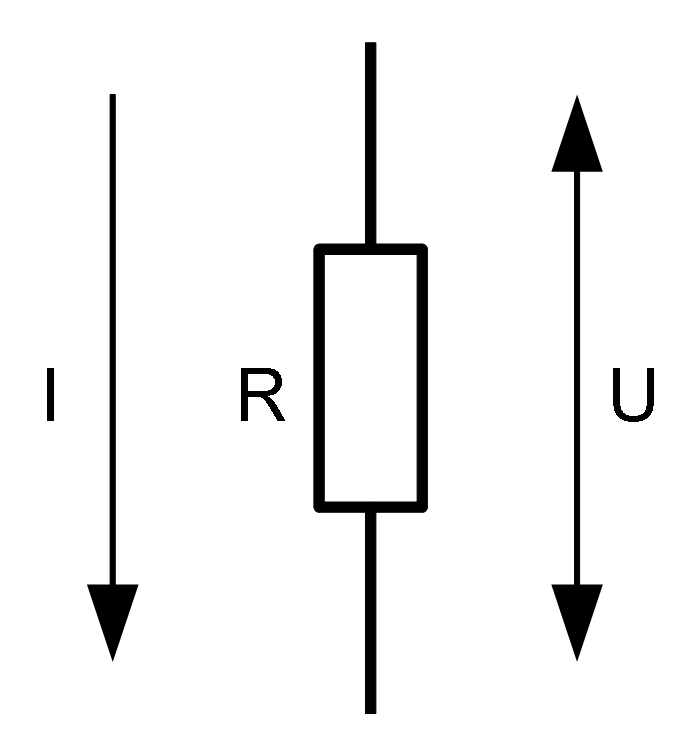# Ohm's Law

Ohm's gk'dfkgdafk; The equation is as follows:Resistance of the conductor, applied voltage and current through the resistance

I = U / R

where:

• I is current
• U is voltage
• R is resistance

Several other equations that are used often in electronics are derived from Ohm's law.